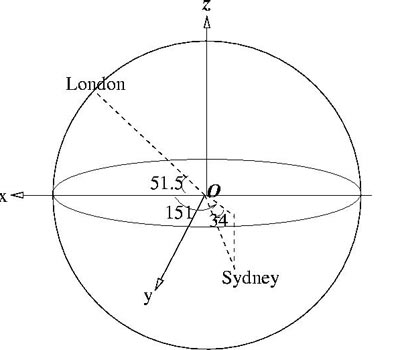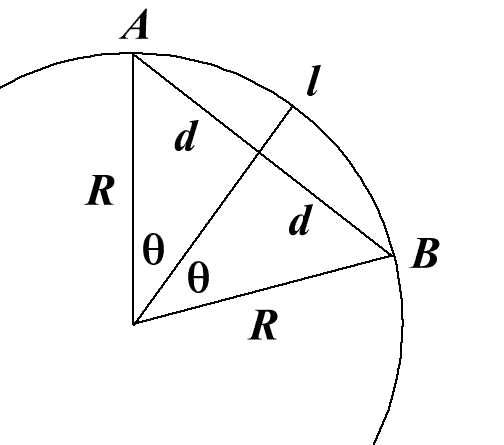#### You may also like### 2D-3D

Two circles of equal size intersect and the centre of each circle is on the circumference of the other. What is the area of the intersection? Now imagine that the diagram represents two spheres of equal volume with the centre of each sphere on the surface of the other. What is the volume of intersection?### The Dodecahedron Explained

What is the shortest distance through the middle of a dodecahedron between the centres of two opposite faces?### When the Angles of a Triangle Don't Add up to 180 Degrees

This article outlines the underlying axioms of spherical geometry giving a simple proof that the sum of the angles of a triangle on the surface of a unit sphere is equal to pi plus the area of the triangle.

# Flight Path

##### Age 16 to 18 Challenge Level:You need to show that if a place has (latitude, longitude) = $(p,q)$ then its coordinates are $$(R \cos p \cos q, R \cos p \sin q, R \sin p).$$Steps in the calculation We need the distance $l$ on the surface between A and B. First calculate the three-dimensional coordinates of A and B from the latitude and longitude of the two points. Then calculate the distance $2d$ (imagine a tunnel straight through the earth from A to B) using Pythagoras Theorem. Use $R$, the radius of the Earth, and $d$ to find the angle $\theta$ radians where $$\sin \theta = {d\over R}.$$ Calculate the arc length $l$.